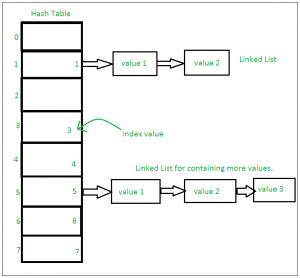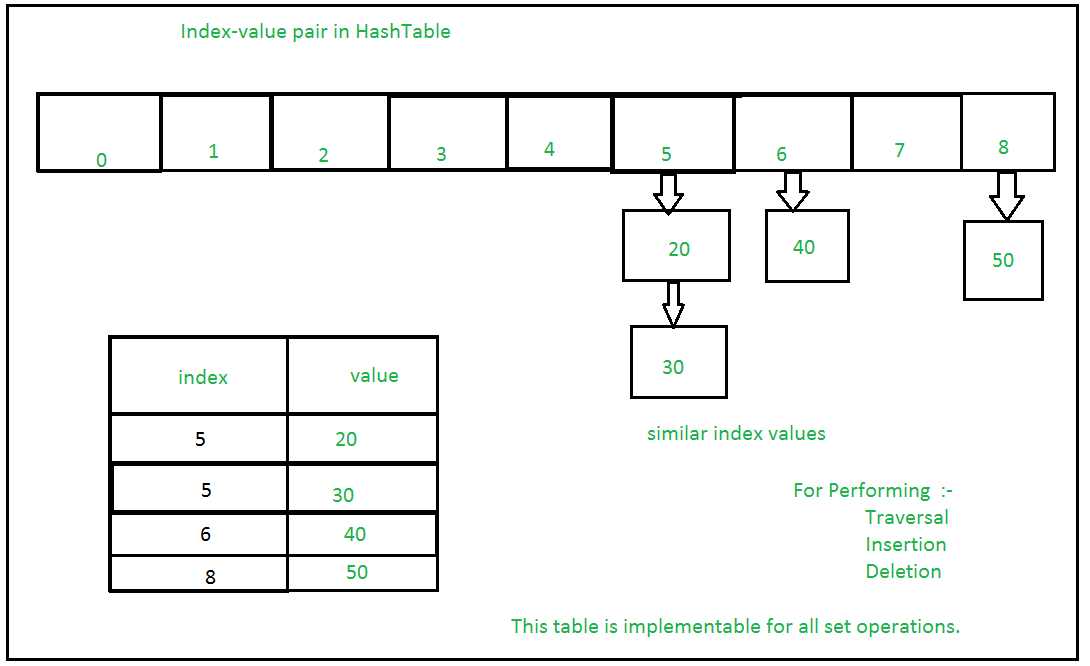Related Articles
Sets in Python
• Difficulty Level : Easy
• Last Updated : 06 Apr, 2020

A Set is an unordered collection data type that is iterable, mutable and has no duplicate elements. Python’s set class represents the mathematical notion of a set. The major advantage of using a set, as opposed to a list, is that it has a highly optimized method for checking whether a specific element is contained in the set. This is based on a data structure known as a hash table. Since sets are unordered, we cannot access items using indexes like we do in lists.

 `# Python program to ` `# demonstrate sets ` ` `  `# Same as {"a", "b", "c"} ` `myset ``=` `set``([``"a"``, ``"b"``, ``"c"``]) ` `print``(myset) ` ` `  `# Adding element to the set ` `myset.add(``"d"``) ` `print``(myset) `

Output:

```{'c', 'b', 'a'}
{'d', 'c', 'b', 'a'}
```

## Frozen Sets

Frozen sets in Python are immutable objects that only support methods and operators that produce a result without affecting the frozen set or sets to which they are applied. While elements of a set can be modified at any time, elements of the frozen set remain the same after creation.
If no parameters are passed, it returns an empty frozenset.

 `# Python program to demonstrate differences ` `# between normal and frozen set ` ` `  `# Same as {"a", "b","c"} ` `normal_set ``=` `set``([``"a"``, ``"b"``,``"c"``]) ` ` `  `print``(``"Normal Set"``) ` `print``(normal_set) ` ` `  `# A frozen set ` `frozen_set ``=` `frozenset``([``"e"``, ``"f"``, ``"g"``]) ` ` `  `print``(``"\nFrozen Set"``) ` `print``(frozen_set) ` ` `  `# Uncommenting below line would cause error as ` `# we are trying to add element to a frozen set ` `# frozen_set.add("h") `

Output:

```Normal Set
set(['a', 'c', 'b'])

Frozen Set
frozenset(['e', 'g', 'f'])
```

## Internal working of Set

This is based on a data structure known as a hash table.
If Multiple values are present at the same index position, then the value is appended to that index position, to form a Linked List. In, Python Sets are implemented using dictionary with dummy variables, where key beings the members set with greater optimizations to the time complexity.

Set Implementation:-Sets with Numerous operations on a single HashTable:-## Methods for Sets

Insertion in set is done through set.add() function, where an appropriate record value is created to store in the hash table. Same as checking for an item, i.e., O(1) on average. However, in worst case it can become O(n).

 `# A Python program to ` `# demonstrate adding elements ` `# in a set ` ` `  `# Creating a Set ` `people ``=` `{``"Jay"``, ``"Idrish"``, ``"Archi"``} ` ` `  `print``(``"People:"``, end ``=` `" "``) ` `print``(people) ` ` `  `# This will add Daxit ` `# in the set ` `people.add(``"Daxit"``) ` ` `  `# Adding elements to the ` `# set using iterator ` `for` `i ``in` `range``(``1``, ``6``):  ` `    ``people.add(i)  ` ` `  `print``(``"\nSet after adding element:"``, end ``=` `" "``) ` `print``(people) `

Output:

```People: {'Idrish', 'Archi', 'Jay'}

Set after adding element: {1, 2, 3, 4, 5, 'Idrish', 'Archi', 'Jay', 'Daxit'}
```

#### Union

Two sets can be merged using union() function or | operator. Both Hash Table values are accessed and traversed with merge operation perform on them to combine the elements, at the same time duplicates are removed. Time Complexity of this is O(len(s1) + len(s2)) where s1 and s2 are two sets whose union needs to be done.

 `# Python Program to  ` `# demonstrate union of ` `# two sets ` ` `  `people ``=` `{``"Jay"``, ``"Idrish"``, ``"Archil"``} ` `vampires ``=` `{``"Karan"``, ``"Arjun"``} ` `dracula ``=` `{``"Deepanshu"``, ``"Raju"``} ` ` `  `# Union using union() ` `# function ` `population ``=` `people.union(vampires) ` ` `  `print``(``"Union using union() function"``) ` `print``(population) ` ` `  `# Union using "|" ` `# operator ` `population ``=` `people|dracula ` ` `  `print``(``"\nUnion using '|' operator"``) ` `print``(population) `

Output:

```Union using union() function
{'Karan', 'Idrish', 'Jay', 'Arjun', 'Archil'}

Union using '|' operator
{'Deepanshu', 'Idrish', 'Jay', 'Raju', 'Archil'}
```

#### Intersection

This can be done through intersection() or & operator. Common Elements are selected. They are similar to iteration over the Hash lists and combining the same values on both the Table. Time Complexity of this is O(min(len(s1), len(s2)) where s1 and s2 are two sets whose union needs to be done.

 `# Python program to  ` `# demonstrate intersection ` `# of two sets ` ` `  `set1 ``=` `set``() ` `set2 ``=` `set``() ` ` `  `for` `i ``in` `range``(``5``): ` `    ``set1.add(i) ` ` `  `for` `i ``in` `range``(``3``,``9``): ` `    ``set2.add(i) ` ` `  `# Intersection using ` `# intersection() function ` `set3 ``=` `set1.intersection(set2) ` ` `  `print``(``"Intersection using intersection() function"``) ` `print``(set3) ` ` `  `# Intersection using  ` `# "&" operator ` `set3 ``=` `set1 & set2 ` ` `  `print``(``"\nIntersection using '&' operator"``) ` `print``(set3) `

Output:

```Intersection using intersection() function
{3, 4}

Intersection using '&' operator
{3, 4}
```

#### Difference

To find difference in between sets. Similar to find difference in linked list. This is done through difference() or – operator. Time complexity of finding difference s1 – s2 is O(len(s1))

 `# Python program to  ` `# demonstrate difference ` `# of two sets ` ` `  `set1 ``=` `set``() ` `set2 ``=` `set``() ` ` `  `for` `i ``in` `range``(``5``): ` `    ``set1.add(i) ` ` `  `for` `i ``in` `range``(``3``,``9``): ` `    ``set2.add(i) ` ` `  `# Difference of two sets ` `# using difference() function ` `set3 ``=` `set1.difference(set2) ` ` `  `print``(``" Difference of two sets using difference() function"``) ` `print``(set3) ` ` `  `# Difference of two sets ` `# using '-' operator ` `set3 ``=` `set1 ``-` `set2 ` ` `  `print``(``"\nDifference of two sets using '-' operator"``) ` `print``(set3) `

Output:

```Difference of two sets using difference() function
{0, 1, 2}

Difference of two sets using '-' operator
{0, 1, 2}
```

#### Clearing sets

`Clear()` method empties the whole set.

 `# Python program to ` `# demonstrate clearing  ` `# of set ` ` `  `set1 ``=` `{``1``,``2``,``3``,``4``,``5``,``6``} ` ` `  `print``(``"Initial set"``) ` `print``(set1) ` ` `  `# This method will remove ` `# all the elements of the set ` `set1.clear() ` ` `  `print``(``"\nSet after using clear() function"``) ` `print``(set1) `

Output:

```Initial set
{1, 2, 3, 4, 5, 6}

Set after using clear() function
set()
```

However, there are two major pitfalls in Python sets:

1. The set doesn’t maintain elements in any particular order.
2. Only instances of immutable types can be added to a Python set.

## Time complexity of Sets

Operation Average case Worst Case notes
x in s O(1) O(n)
Union s|t O(len(s)+len(t))
Intersection s&t O(min(len(s), len(t)) O(len(s) * len(t)) replace “min” with “max” if t is not a set
Multiple intersection s1&s2&..&sn (n-1)*O(l) where l is max(len(s1),..,len(sn))
Difference s-t O(len(s))

## Operators for Sets

Sets and frozen sets support the following operators:

Operators Notes
key in s containment check
key not in s non-containment check
s1 == s2 s1 is equivalent to s2
s1 != s2 s1 is not equivalent to s2
s1 <= s2 s1 is subset of s2
s1 < s2 s1 is proper subset of s2
s1 >= s2 s1 is superset of s2
s1 > s2 s1 is proper superset of s2
s1 | s2 the union of s1 and s2
s1 & s2 the intersection of s1 and s2
s1 – s2 the set of elements in s1 but not s2
s1 ˆ s2 the set of elements in precisely one of s1 or s2

Code Snippet to illustrate all Set operations in Python

 `# Python program to demonstrate working# of ` `# Set in Python ` ` `  `# Creating two sets ` `set1 ``=` `set``() ` `set2 ``=` `set``() ` ` `  `# Adding elements to set1 ` `for` `i ``in` `range``(``1``, ``6``): ` `    ``set1.add(i) ` ` `  `# Adding elements to set2 ` `for` `i ``in` `range``(``3``, ``8``): ` `    ``set2.add(i) ` ` `  `print``(``"Set1 = "``, set1) ` `print``(``"Set2 = "``, set2) ` `print``(``"\n"``) ` ` `  `# Union of set1 and set2 ` `set3 ``=` `set1 | set2``# set1.union(set2) ` `print``(``"Union of Set1 & Set2: Set3 = "``, set3) ` ` `  `# Intersection of set1 and set2 ` `set4 ``=` `set1 & set2``# set1.intersection(set2) ` `print``(``"Intersection of Set1 & Set2: Set4 = "``, set4) ` `print``(``"\n"``) ` ` `  `# Checking relation between set3 and set4 ` `if` `set3 > set4: ``# set3.issuperset(set4) ` `    ``print``(``"Set3 is superset of Set4"``) ` `elif` `set3 < set4: ``# set3.issubset(set4) ` `    ``print``(``"Set3 is subset of Set4"``) ` `else` `: ``# set3 == set4 ` `    ``print``(``"Set3 is same as Set4"``) ` ` `  `# displaying relation between set4 and set3 ` `if` `set4 < set3: ``# set4.issubset(set3) ` `    ``print``(``"Set4 is subset of Set3"``) ` `    ``print``(``"\n"``) ` ` `  `# difference between set3 and set4 ` `set5 ``=` `set3 ``-` `set4 ` `print``(``"Elements in Set3 and not in Set4: Set5 = "``, set5) ` `print``(``"\n"``) ` ` `  `# checkv if set4 and set5 are disjoint sets ` `if` `set4.isdisjoint(set5): ` `    ``print``(``"Set4 and Set5 have nothing in common\n"``) ` ` `  `# Removing all the values of set5 ` `set5.clear() ` ` `  `print``(``"After applying clear on sets Set5: "``) ` `print``(``"Set5 = "``, set5) `

Output:

```('Set1 = ', set([1, 2, 3, 4, 5]))
('Set2 = ', set([3, 4, 5, 6, 7]))

('Union of Set1 & Set2: Set3 = ', set([1, 2, 3, 4, 5, 6, 7]))
('Intersection of Set1 & Set2: Set4 = ', set([3, 4, 5]))

Set3 is superset of Set4
Set4 is subset of Set3

('Elements in Set3 and not in Set4: Set5 = ', set([1, 2, 6, 7]))

Set4 and Set5 have nothing in common

After applying clear on sets Set5:
('Set5 = ', set([]))
```

Recent articles on Python Set.

This article is contributed by Jay Patel. If you like GeeksforGeeks and would like to contribute, you can also write an article and mail your article to contribute@geeksforgeeks.org. See your article appearing on the GeeksforGeeks main page and help other Geeks.